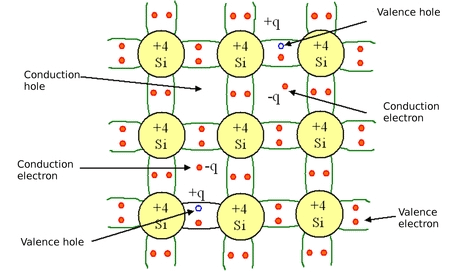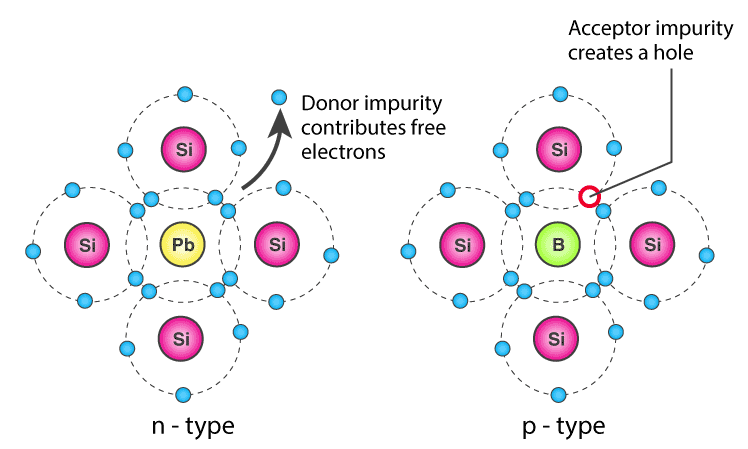Courses

# Conductivity Of Semiconductor - Practice GATE Notes | EduRev

## GATE : Conductivity Of Semiconductor - Practice GATE Notes | EduRev

The document Conductivity Of Semiconductor - Practice GATE Notes | EduRev is a part of GATE category.
All you need of GATE at this link: GATE

Conductivity of Semiconductors

➢ Conductivity in Intrinsic Semiconductor

• In a pure semiconductor, the number of holes is equal to the number of electrons. Thermal agitation continues to produce new electron-hole pairs and these electron-hole pairs disappear because of recombination.Conductivity in Intrinsic Semiconductors

• With each electron-hole pair created, two charge-carrying particles are formed. One is negative which is a free electron with mobility µn. The other is a positive i.e., hole with mobility µp. The electrons and hole move in the opposite direction in an electric field E, but since they are of opposite sign, the current due to each is in the same direction.
• Hence the total current density J within the intrinsic semiconductor is given by:
J = Jn + Jp
= q.n.µn.E + q.p.µp.E
= (n.µn + p.µp)qE
= σ.E
where n=no. of electrons/unit volume i.e., the concentration of free electrons
p= no. of holes/unit volume i.e., the concentration of holes
E=applied electric field strength, V/m
q= charge of electron or hole in Coulombs
• Hence, σ is the conductivity of semiconductor which is equal to (n µn + p µp)q. The resistivity of semiconductor is reciprocal of conductivity.
Ρ = 1/σ
• It is evident from the above equation that current density within a semiconductor is directly proportional to applied electric field E.
• For a pure semiconductor, n = p = ni
where ni = intrinsic concentration.
The value of ni  is given by:
ni= ATexp (-EGO/KT)
therefore,  J = nin + µp)qE
• Hence, conductivity in an intrinsic semiconductor is: σ= nin +  µp)q
• Intrinsic conductivity increases at the rate of 5% per oC for Ge and 7% per o C for Si.

➢ Conductivity in Extrinsic Semiconductor (N-Type  and P-Type)

• The conductivity of intrinsic semiconductor is given by:
σ= nin + µp)q
= (n.µn + p.µp)q
• For N-type , n>>p
Therefore, σ = q.n.µn
• For P-type ,p>>n
Therefore, σ = q.p.µpExtrinsic Semiconductors

Charge Densities in P-Type and N-Type Semiconductor

➢ Mass Action Law

• Under thermal equilibrium for any semiconductor, the product of the no. of holes and the concentration of electrons is constant and is independent of the amount of donor and acceptor impurity doping.
n.p = ni2
where n= electron concentration
p = hole concentration
ni= intrinsic concentration
• In N-type semiconductor as the no. of electrons increase, the no. of holes decreases. Similarly in P-type, as the no. of holes increases the no. of electrons decreases. Thus, the product is constant and is equal to ni2 in case of intrinsic as well as extrinsic semiconductor.
• The law of mass action has given the relationship between free electrons concentration and hole concentration. These concentrations are further related by the law of electrical neutrality, as explained below.

➢ Law of Electrical Neutrality

• Semiconductor materials are electrically neutral. According to the law of electrical neutrality, in an electrically neutral material, the magnitude of positive charge concentration is equal to that of negative charge concentration.
• Let us consider a semiconductor that has ND donor atoms per cubic centimetre and NA acceptor atoms per cubic centimetre i.e., the concentration of donor and acceptor atoms are ND and NA, respectively.
• Therefore, ND positively charged ions per cubic centimetre are contributed by donor atoms and NA negatively charged ions per cubic centimetre are contributed by the acceptor atoms. Let n, p be concentration of free electrons and holes respectively. Then according to the law of neutrality,
ND + p = NA + n   ...eq 1.1
For N-type semiconductor, N= 0 and n>>p.
Therefore, ND ≈ n ...eq 1.2
• Hence for N-type semiconductor, the free electron concentration is approximately equal to the concentration of donor atoms. In later applications since some confusion may arise as to which type of semiconductor is under consideration at the given moment, the subscript n or p  is added for N-type or P-type respectively. Hence eq 1.2 becomes ND ≈ nn.
• Therefore, the current density in N-type semiconductor is J = NDn.q.E
and conductivity σ = NDn.q
• For P-type semiconductor,
N= 0 and p>>n.
Therefore NA ≈ p or NA ≈ pp
• Hence for P-type semiconductor, the hole concentration is approximately equal to the concentration of acceptor atoms.
Current density in N-type semiconductor is J = NAp.q.E
And conductivity σ = NAp.q
• Mass action law for N-type
nn.p= ni2
p= ni2/ND
since (n≈ ND)
• Mass action law for P-type
np.p= ni2
n= ni2/ NA
since (p≈ NA)
Offer running on EduRev: Apply code STAYHOME200 to get INR 200 off on our premium plan EduRev Infinity!

,

,

,

,

,

,

,

,

,

,

,

,

,

,

,

,

,

,

,

,

,

;